/

### Function Description

Returns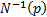, the inverse of the unit normal cumulative distribution function. This is the valuefor which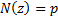where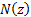is the cumulative unit Normal distribution function, see MnCumulativeNormal. The function is not defined if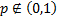.

In practice, the website uses a rational approximation to the above function developed by Acklam (2005). This is not the same approach used in some versions of Microsoft Excel which appear to calculate this function using an iterative search method based on the methodology Microsoft use to calculate. Otherwise the equivalent function in Microsft Excel is NORMSINV.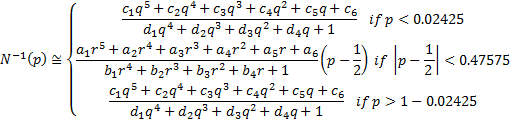where: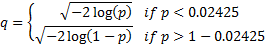and: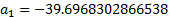,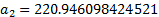,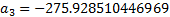,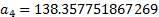,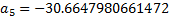,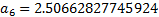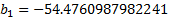,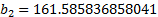,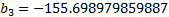,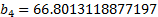,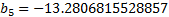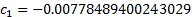,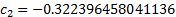,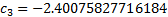,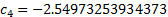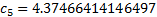,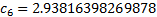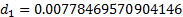,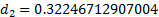,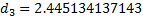,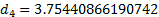Contents | Prev | Next